Next: Learning: Conditional vs. Joint Up: Temporal Modeling Previous: Pre-Processing Implementation and Exponential

# Probabilistic Time Series Modeling

Of course, immediately after the time window over the past, another observation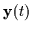(of the near future) is also obtained from the training data. One may again simply vectorize the parameters of the perceptual system (the Gaussian tracking blobs) into yet another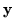vector (of dimensionality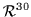). The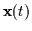vector represents the past action and therepresents the consequent reaction exactly at time t. For a few minutes of data, we can obtain thousands of pairs of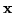andvectors (i.e. action-reaction pairs) by sliding the attentional window over the time series (i.e. considering all values of t) and processing as explained above. Figure 4.5 shows the evolution of the dominant 3 dimensions of thevectors as we consider an involved interaction between two participants over time t of roughly half a minute. This represents the evolution of the short term memory of the learning system during half a minute.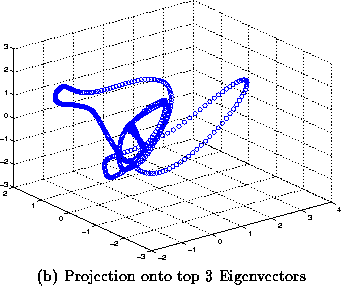Given sufficient pairs of the vectors ((t),(t)) from training data, it is possible to start seeing patterns between a short term memory of the past interaction of two humans and the immediate subsequent future reaction. A system which can forecast this behaviour could predict what to do next and engage with a single human. However, instead of learning an exact deterministic mapping betweenand, as is done in a predictive neural network, we will discuss a more probabilistic approach. This involves estimating a probability density denoted as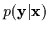which yields the probability of a reaction given a short history of past action. We will always be observing the past () but the future () is what we are trying to predict. We are not, for instance, interested in the conditional pdf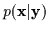, which computes the probability of the past () given the future. In other words, we will seldom use the learning system in the almost `philosophical' task of describing the actions that could have led to some future result,. Mostly, we will query the system about what future result should follow the actions it just saw. The use of probabilistic techniques here allows the notion of randomness and stochasticity which are more appropriate for synthesizing compelling behaviour. In essence, they make the system generate behaviour that is interesting and correlated with the past and the user's stimulating actions but is also not entirely predictable and contains some pseudo random choices in its space of valid responses.Next: Learning: Conditional vs. Joint Up: Temporal Modeling Previous: Pre-Processing Implementation and Exponential
Tony Jebara
1999-09-15